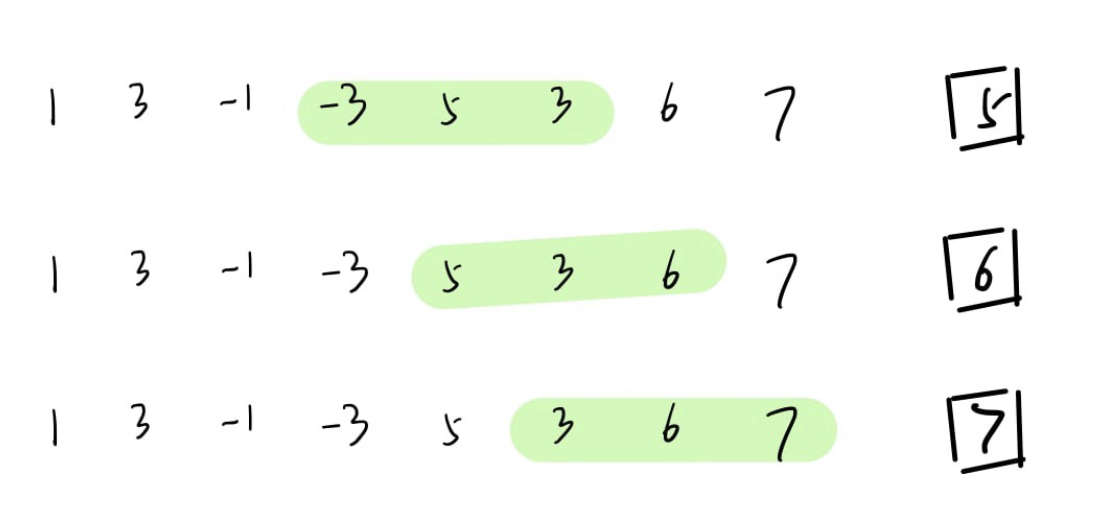# Sliding Window Maximum Problem is Not that Difficult

## Three approaches to solving the sliding window maximum problemSliding Window Maximum Problem

# Problem Statement

`Input: nums = [1,3,-1,-3,5,3,6,7], k = 3Output: [3,3,5,5,6,7]Explanation: Window position                Max---------------               -----[1  3  -1] -3  5  3  6  7       3 1 [3  -1  -3] 5  3  6  7       3 1  3 [-1  -3  5] 3  6  7       5 1  3  -1 [-3  5  3] 6  7       5 1  3  -1  -3 [5  3  6] 7       6 1  3  -1  -3  5 [3  6  7]      7`

# Solution 1: Brute Force

`def simple(nums, k):   res = []   length = len(nums)           i = 0   while i < length-k+1:     mx = max(nums[i:i+k])     res.append(mx)     i +=1                     return res`

# Solution 2: Priority Queue

`import heapqclass Solution:    def maxSlidingWindow(self, nums, k):        lens = len(nums)        h  = []        res = []                for r in range(k):            heapq.heappush(h, (-nums[r],r))        res.append(-h)                        for r in range(k, lens):            l = r-k+1            # window index range: l, l+1, ..., l+k-1            heapq.heappush(h, (-nums[r],r))            while h <l or h > l+k-1:                heapq.heappop(h)            res.append(-h)                return res`

# Solution 3: Monotonic Deque

`from collections import dequeclass Solution:    def maxSlidingWindow(self, nums, k):        res = []                d = deque([])                for r in range(len(nums)):             # remove item outside the window            #  1. if first index in  Q(Deque) < i-k+1            if d and d < r-k+1:                d.popleft()                        #  2. a new bigger item comes            while d and nums[r] >= d:                d.popleft()                        # keep Monotonic            while d and nums[r] > d[-1]:                d.pop()                            d.append((nums[r],r))            if r >= k-1:                res.append(d)                return res`

Developer in China, AI and machine learning enthusiast

## More from Jerry An

Developer in China, AI and machine learning enthusiast